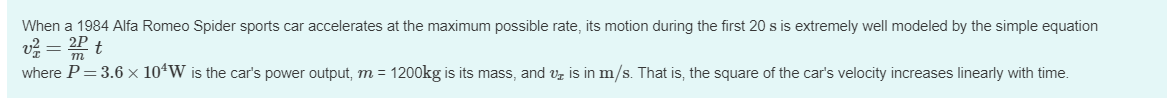# When a 1984 Alfa Romeo Spider sports car accelerates at the maximum possible rate, its motion during the first 20 s is extremely well modeled by the simple equationuz 2Ptwhere P 3.6 × 104w is the car's power output, m : 1200 g is its mass, and s in m s. That is, the square of the cars velocity increases i early th time

Question

The prompt is in the image attached. The questions I need help are:

a) Evaluate the acceleration at t=1.0 s. Express your answer in m/s^2 in 2 significant figures.
b) Evaluate the acceleration at t=11s. Express your answer in m/s^2 in 2 significant figures.

Thank you!help_outlineImage TranscriptioncloseWhen a 1984 Alfa Romeo Spider sports car accelerates at the maximum possible rate, its motion during the first 20 s is extremely well modeled by the simple equation uz 2Pt where P 3.6 × 104w is the car's power output, m : 1200 g is its mass, and s in m s. That is, the square of the cars velocity increases i early th time fullscreen
check_circle

star
star
star
star
star
1 Rating
Step 1

As per the question, the motion is given by the equation

Step 2

Acceleration is given as time rate of change of velocity. hence taking derivative of the velocity equation both side relative to "t", we get

Step 3

(a)

Acceleration at t = 1.0 s is given as

Hence the acceleration comes out to be 3...

### Want to see the full answer?

See Solution

#### Want to see this answer and more?

Solutions are written by subject experts who are available 24/7. Questions are typically answered within 1 hour.*

See Solution
*Response times may vary by subject and question.
Tagged in

### Kinematics